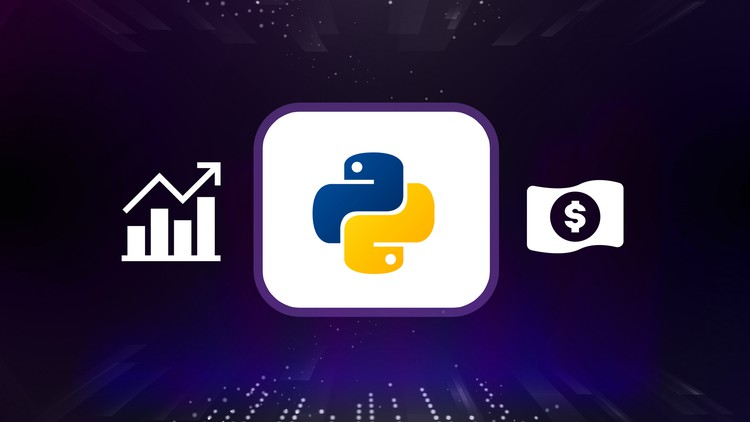# Basic Finance with PythonLearn the basics of finance with python through a learning by doing model

What you will learn

### Create graphics to visualize the impacts of different parameters on financial models

Description

Have you ever wondered what the different financial metrics and models mean? This course will give you an introduction to the very exciting world of finance through the lens of programming in python. You can expect to both increase your python skills while also building your financial acumen.

Some of the course deals with how cash flows compound and how they are discounted for present value. Other lectures focus on the models that are used for evaluating fixed income investments and equity investments. All lectures are done through jupyter notebooks with python code to replicate different equations, visualize the evolution of financial models and explain with examples how the different pieces of financial theory fit together.

The structure of the course is:

Compound Interest: Lectures on how compound interest works

Present Value: Lectures on how to compute the present value of different streams of cash flows

Annuities: Lectures on how to value annuity type payment streams

Perpetuities: Lectures on what a perpetuity and how one might value infinite streams of cash flows

Bonds: Lectures on how to value bond cash flows

Dividend Discount Model: Lectures on the dividend discount model which is used in valuing stocks

Risk: Lectures on how risk is measured and how diversification can reduce risk

Capital Asset Pricing Model: Lectures on the very famous Capital Asset Pricing Model and how it is used for finding the expected returns of stocks.

English
language

Content

Introduction

### Compound Interest Part 1

Compound Interest Introduction
Comparing Compound Interest Curves

### Compound Interest Part 2

Changing Rates
Cumprod Function
Withdrawal Effects
Timing Rates for Withdrawals
Annualizing Rates
Solving for Other Variables

### Present Value

Introduction
Discounting a Cash Flow
Judging Investment Opportunities
Present Value Outside Endpoints
Future Value

### Annuities

Annuity Introduction
Annuity and Annuity Factor Equation
Annuity Factor Details
Annuity Due
Semi-annual Payments
Difference Between Annualized and Nominal Rate
Converting to an Annualized Rate
Extending to Four Payments

### Perpetuities

Payment Values
Tracking Cumulative Value
Perpetuity Equation

### Bonds

Bond Intro Part 1
Bond Intro Part 2
Bond Equation & Par Bonds
Discount/Premium Bonds & Pull to Par
Solving for the Coupon Rate
Solving for r

### Dividend Discount Model

Introduction
The Impact of Growth
Dividend Discount Model with Growth
Dividend Yield

### Risk

Risk Intro & Holding Period Return
Arithmetic and Geometric Mean
Variance and Standard Deviation
Annualizing Returns
Annualizing Variance and Standard Deviation
Covariance Introduction
Covariance Equation
Correlation
Beta
Systematic and Idiosyncratic Risk
Simulating 100 Stocks
Diversification

### Capital Asset Pricing Model

CAPM Introduction
Comparing CAPM
Stocks in CAPM
Portfolio Beta
Shorting a Stock
Shorting Example
Extended CAPM Graph
Alpha
Profit with Alpha